Science Journal of Applied Mathematics and Statistics
Volume 5, Issue 1, February 2017, Pages: 1-9

Mixed Seasonal and Subset Fourier Model with Seasonal Harmonics

Iberedem Aniefiok Iwok, Murphy Dooga

Department of Mathematics/Statistics, University of Port-Harcourt, Port Harcourt, Nigeria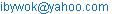(I. A. Iwok)

Iberedem Aniefiok Iwok, Murphy Dooga. Mixed Seasonal and Subset Fourier Model with Seasonal Harmonics. Science Journal of Applied Mathematics and Statistics. Vol. 5, No. 1, 2017, pp. 1-9. doi: 10.11648/j.sjams.20170501.11

Received: September 30, 2016; Accepted: October 13, 2016; Published: January 14, 2017

Abstract: In this work, Box-Jenkins seasonal model was fitted to a temperature series and the assumption of model adequacy was found to be violated. Subset Fourier series with seasonal harmonics was introduced and added to the pure seasonal component that was found to be inadequate. This combination resulted in a mixed seasonal and subset Fourier model with seasonal harmonics. The mixed model was fitted to the data and was subjected to diagnostic checks. The tests revealed that the model was adequate. Comparative study was also carried out and the results showed that the mixed model performed better than the pure seasonal and the subset Fourier model.

Keywords: Seasonal Model, Fourier Series, Subset Fourier Series, Model Selection, Periodogram and White Noise Process

1. Introduction

Climate change has immense effects on business and economic activities. As a result, forecasts of climate change that are accurate and cost effective are crucial to effective managerial decision-making Thus, the operations and strategic decision-making in these activities need to take into account the realised and potential effects of climate change.

Historical trends in climatic variables (temperature, rainfall, humidity etc.) are of special interest in diverse academic disciplines and economic sectors such as agriculture, ecology, water resource management etc. As a result, numerous studies have investigated climatic trends from station records of variables like temperature and rainfall as well as various indices derived from these quantities. These station records made with respect to time are referred to as time series. For the purpose of this study, we shall be considering temperature as one of the key indicators of climate change.

Temperature is said to be the degree of hotness and coldness of a substance measured on some definite scale. Hotness and coldness result from molecular activities. As molecules take up energy, they start to move faster and the temperature of the substances increases. Thus, it could be posit that temperature is a measure of the average kinetic energy of the molecules of a substance. It is therefore, a means of determining the internal energy contained within a system.

Due to the fact that human beings instantly perceive the amount of heat and cold within an area, it is understandable that temperature is a feature of reality that we have a fairly intuitive grasp on. It might interest us to note that, temperature is different from heat, though the two concepts are linked. While temperature is a measure of the internal energy of the system, heat on the other hand is a measure of how energy is transferred from one system or body to another. Hence, the greater the heat absorbed by a material or system, the more rapidly the atoms within the material begin to move and thus the greater the rise in temperature.

The temperature of a system or body is measured by a thermometer. A traditional thermometer measures temperature by a fluid that expands as it gets hotter and contracts as it gets cooler. As the temperature changes, the liquid within the tube moves along a scale on the temperature device. On planet earth, the climatic condition of a particular place can be detected by the degree of temperature rise or fall. This degree of temperature can be recorded with respect to time in form of a series. Such data collected over time forms a ‘time series’.

A time series is a collection of set of observations or data, generated or recorded over a period of time. This time could be hourly, weekly, monthly, quarterly, yearly etc. An analysis of time series can be used to make current and future decisions and plans based on long-term forecasting. Usually, assumptions are made that past patterns will continue into the future.

Some time series are periodic in nature and these periodicities may be of interest. A common technique used to study periodic data is Fourier analysis.

In time series, periodicities are found by looking for sharp peaks when searching the "standard" periodogram from Fourier analysis. These peaks usually correspond to intrinsic periodicities in the time series. In Fourier analysis approach, time series can be expressed as a combination of cosine or sine waves with differing periods and amplitudes. This fact can be utilized to examine the periodic behaviour in a time series.

A periodic function repeats its behaviours at regular intervals or periods. The most common examples are the trigonometric functions, which repeat over intervals of 2π radians.

A way of analyzing a time series is based on the assumption that it is made up of sine and cosine waves with different frequencies. A device that uses this idea is the periodogram first introduced by Schuster in 1898 and modified by several researchers of the present era. The periodogram is commonly used for identifying the dominant cyclical behaviour in a series, most especially when the cycles are not related to the commonly encountered seasonalities. Of course, the identification of the periodicities of a time dependent variable is important in prediction theory and hazards prevention.

As a result of the effect of global warming on earth, researches are highly encouraged on climate indices such as humidity, rainfall, temperature etc. Since these variables are periodic time varying quantities, it is obvious that the modelling structure of these quantities follow the Fourier approach. However, the basic intension of this work is to reduce the computational burden usually encountered in fitting the complete Fourier model and to focus on ‘temperature’ variable only. This is going to be achieved by employing a method of reducing the number of Fourier terms involved and combining it with Box-Jenkins seasonal model. Comparative study will be carried out to see how best this new approach fit the data better than the usual cumbersome Fourier model.

2. Literature Review

A lot of works have been done in search of solutions to some of man’s climate problems.

 used Fourier series method to model the mean monthly temperature of Uyo metropolis. The Model was adjudged to be statistically significant and fitted well to the data when a test of significance of the general model and the overall goodness of fit was administered.

 modelled the properties of global mean temperature data-set using Univariate time series techniques. The analysis resulted in developing a parsimonious forecasting model and the forecast evaluation showed that the chosen model performed well against the rival models.

 examined a large data set involving more than 50 years of rainfall and temperature data using spectral analysis. In the research, the interactions between the two variables were examined. The rainfall data was found to appear seasonal while the temperature data appeared stationary. The analysis revealed a cycle of 2-3years and an inverse relationship in trend between rainfall and daily temperature range.

 modelled monthly Inflation rates in Nigeria from 2011-2013 using periodogram and Fourier series technique. An inflation cycle of 51 months was discovered and a Fourier series model equivalent to this period was fitted to the data. Forecasts of 13 months inflation rate were generated and the model was found to give good estimates of the actual values.

 in their research compared the classical spectrum estimation method, simulated periodogram method, Barlette method and Welch method of power spectrum estimation. Spectral resolution of different length of data was considered. The spectral estimation and variance method revealed that the Welch method performs better than other methods in periodic series.

 used seasonal autoregressive integrated moving average with exogenous variables (SARIMAX) and an artificial neural network (ANN) models to forecast hourly temperature of electricity load data in Mainand of Abu Dhabi. It was found that the ANN model produced more accurate temperature predictions than the SARIMAX model. Pre-whitening method was used to determine the lagged effect of temperature of the electricity load. Root mean square error (RMSE) and the mean absolute percentage error (MAPE) were used to evaluate the comparison between the two models. The study showed that the SARIMAX model behaved better at estimation stage but worst at forecasting stage.

 used mean values and variances of the estimated deterministic seasonal cycles to standardize the Japan Meteorological Agency (JMA) daily mean surface temperature data. A parametric form of a non-stationary autoregressive (AR) model and an ordinary AR were considered to quantify the anomalies in the data. It was found that the parametric form of a non-seasonal AR model fitted substantially better and exhibited a significant seasonal structure in their auto-correlation than the ordinary AR. The non-seasonal model also performed better in determining the climatic influence on anomalies of surface air temperature in Japan when it was applied to a high-pass filtered data to investigate the relationship between the seasonal structure and high frequency variability in anomalies.

 modelled the daily average mean temperature of Sokoto metropolis using autoregressive fractional integrated moving average. It was discovered that ARFIMA (3, 0.6238841, 1) was the best optimal model that can best forecast the Sokoto metropolis temperature.

 used SARIMA (0, 0, 0)*(2, 1, 0)12 model to forecast rainfall amount in Ashanti region of Ghana. It was found that rainfall in Ashanti region significantly changes over time. Periods of low variability and of extreme variability separated by periods of transition were also found and forecast figures for some of the months showed an increase in the rainfall figures for the subsequent years. It was also noted that the model with the least AIC and BIC values during a tentative model test is the best model to be used in such analysis.

 predicted the monthly values of surface Ozone (O3) concentration using autoregressive (AR) of order 1. Assekrem region of Algeria was used as a case study. The Box-Jenkins approach was applied to construct the forecast model. A comparison of the measured O3 concentration values and the forecasted values showed that the model satisfactorily predicted monthly average O3 concentrations.

As noted in the above literature reviews, a lot of periodic and non periodic models have been fitted to the different climatic variables. To avoid duplication of purpose, this work considers a situation where a part of the Fourier series is extracted and added to the Box-Jenkins seasonal model to correct the inefficiency and inadequacy of the seasonal model in cases where they are found inadequate.

3. Methodology

3.1. Differencing

The simplest form of differencing is given by the expression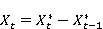(1)

where,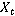is the differenced series and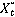is the raw series. The differencing is used to convert a non-stationary series to a stationary process.

3.2. Seasonal Autoregressive Integrated Moving Average Model

Seasonal autoregressive integrated moving average (SARIMA) model is used for time series with periodic and non periodic behaviour. The SARIMA multiplicative model is written as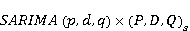(2)

and this can be expressed explicitly as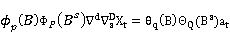(3)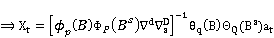(4)

where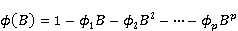,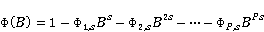,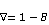,,,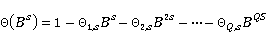,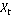is the time series at time,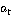is the white noise process,is the season,is the order of autoregressive components,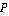is the order of seasonal autoregressive components,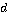is the order of non-seasonal differencing,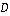is the order of seasonal differencing,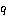is the order of moving average component,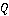is the order of seasonal moving average component.

3.3. Autocovariance and Autocorrelation Function

Given a working series of time series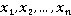; the sample autocovariance (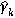) at lag k is given asand the sample autocorrelation (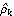) at lag k is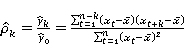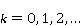(5)

The plot of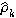versus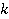is the sample correlogram.

3.4. Model Selection Criterion

These are criteria used for selecting the best model (order of the model) that fit a data. The commonly used ones are:

3.4.1. Akaike’s Information Criteria (AIC)

The AIC is defined as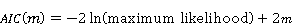where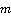is the number of parameters in the model.

3.4.2. Bayesian Information Criteria (BIC)

The BIC is defined as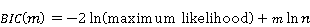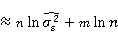whereis the sample size.

The order of the model is chosen so as to minimize the AIC or BIC.

3.5. Fourier Series

Fourier series are infinite series that represent periodic (seasonal) functions in terms of cosines and sines. A Fourier series representation of a function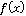over the interval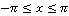is an expression of the form(6)

where the coefficients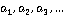and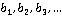are determined by the function.

3.6. White Noise Process

A process {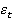} is said to be a white noise process with mean 0 and variance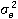written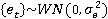if it is a sequence of uncorrelated random variables from a fixed normal distribution.

3.7. Time Series Representation of the Fourier Series

In time series, the infinite sum of the Fourier series in (6) can be approximated by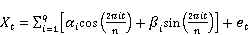(7)

estimated by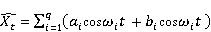(8)

where,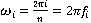,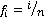is the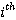harmonic of the fundamental frequency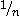,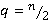is the highest harmonic,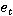is a white noise process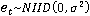. The coefficients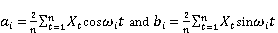.

Observantly, despite the time series approximation of the Fourier series expressed in (7); the approximation is still boring and space consuming when considering large samples.

3.8. The Periodogram

The Periodogram is usually used to reveal the hidden periodicities (seasons) in time series. The Periodogram is the plot of intensities against the frequencies or the periods. It helps in determining the season in time series. This is usually indicated by the largest peak in the Periodogram plot.

The Periodogram function is obtained as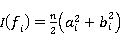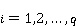(9)

3.9. Subset Fourier Series with Seasonal Harmonics

Instead of settingin (7), the highest harmonic is expressed in terms of the number of seasons,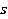. In other words, we set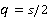, and expressaccordingly. That is,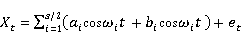(10)

Though this setting (10) may not give accurate fit to the data; our intention is to incorporate (10) into an inadequate seasonal model (3) to see whether it can give model adequacy.

3.10. The Mixed seasonal and Subset Fourier Model with Seasonal

Harmonics

Combining the seasonal model in (4) and the subset Fourier model with seasonal harmonics in (10) results in the following mixed expression: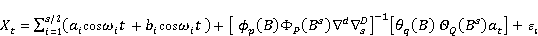(11)

The interest here is to reduce the computational burden in using the complete Fourier time series model in (7) while taking into cognizance the results produced in the end. Of course, it is clear in equation (11) that the number of terms involved is less than that involved in (7). Thus, if equation (11) produces an adequate fit to the data; then a larger part of our major objectives is achieved. The parameters of the mixed seasonal and Fourier model with seasonal harmonics can easily be obtained by subjecting expression (11) to regression analysis and obtaining the errors for further analysis. Since the model (11) is additive, we assume that the pure seasonal components and the Fourier part are uncorrelated.

4. Diagnostic Checks

4.1. Residual Analysis

The diagnostic checks are usually applied to the residuals obtained from the fitted model. The basic assumption is that if the model is adequate, the residuals are expected to resemble a white noise process. The residual is the difference between the actual values and the fitted values. Each residual is the unpredictable component of the associated observation.

The estimated residual is given as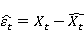(12)

where,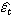is the estimated residual seriesis the actual values (the time series itself)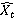are the fitted values.

The residuals are analysed to ensure that the assumptions of the model adequacy are satisfied.

4.2. Residual Autocorrelation Function

Under the null hypothesis that the residuals are serially uncorrelated, the autocorrelation function (ACF) of the residuals obtained from fitting (11) is observed for model adequacy. If the model is adequate, there will be no significant autocorrelation in the autocorrelation plot. An autocorrelation is statistically significant if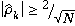. If this is achieved, the null hypothesis is not rejected and the residuals are said to follow a white noise process.

4.3. Actual and Estimate Plots

If the model is adequate, the super imposed plots of the actual and estimate values will reflect a strong correlation and closeness between them.

5. Data Analysis and Results

The data used for this work is the average monthly temperature data (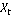) in Markudi, Nigeria between 2006-2015 (Source: www.cbn.gov.ng); and the analysis is carried out using Minitab and gretl softeware.

5.1. Graphical Presentation of Series

An assessment of the graphical presentation of the original series shows that there exist seasonal variations; but the cycle or period of the series cannot be exactly ascertained (see figure 1).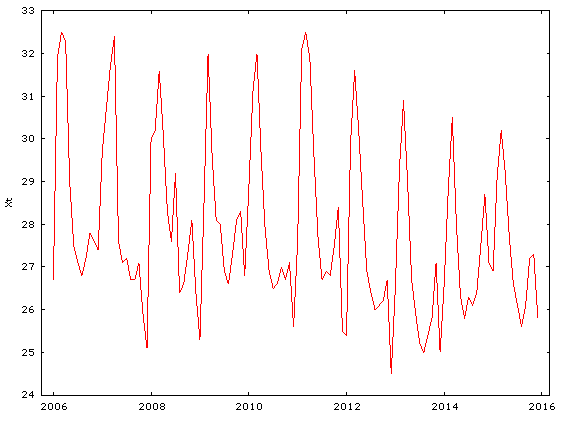Figure 1. Plot of the original series.

In addition, the raw data plot (figure 1) exhibits some elements of non-stationarity. Thus, differencing transformation is required to obtain stationarity.

5.2. Differencing the Original Series

Using the differencing transformation in equation (1), the differenced series is plotted in figure 2 below. Clearly, the series is now stationary and the modelling process can commence.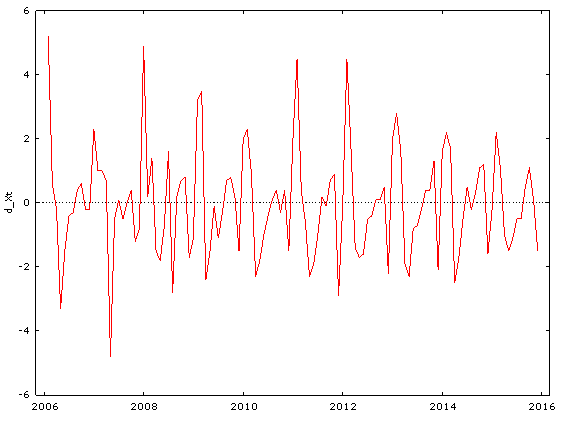Figure 2. Plot of the differenced series.

5.3. The Periodogram

The periodogram plot is displayed in figure 3. The highest peak occurs at a period of 12 with a spectral density of 17.831. This shows that the season,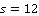.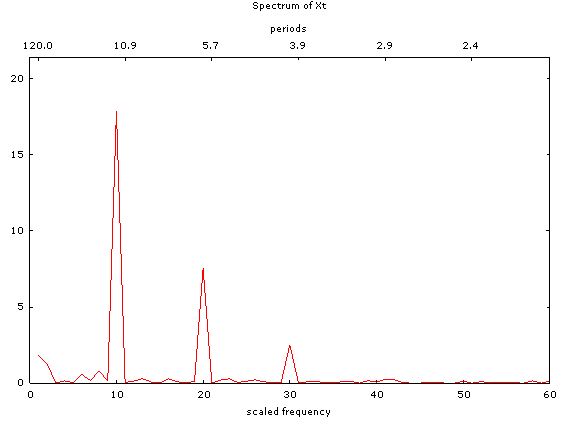Figure 3. The Periodogram plot of the series.

5.4. SARIMA Model Selection

Since a season has been found using the periodogram, it is imperative for a seasonal model to be fitted. The model selection criteria in section 3.5 were applied and the model with the least AIC and BIC is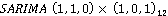and is therefore selected.

5.5. Parameters Estimation

Using (3), the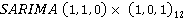model can be explicitly expressed as: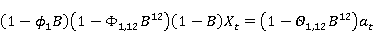(13)

Using the parameter values provided by the gretl software in fitting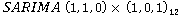, the model (13) can finally be expressed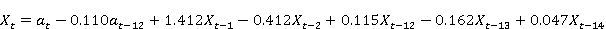(14)

5.6. Diagnostic Check of the SARIMA Model

Though the parameters of the model (14) were found to be statistically significant; the model failed when subjected to diagnostic checks. In the first place, the residuals are not well behaved. This is clearly shown in the plots of the residual ACF (see figure 4 of the appendix). In this plot, there exist significant spike(s) in the ACF plots at lag 24. Hence the residual autocorrelations are serially correlated. This implies that the fitted seasonal model is not adequate. In addition, the residual variance is found to be 7.21.

5.7. Subset Fourier Series with Seasonal Harmonics

The complete Fourier model was expressed in (7). As noted earlier, for a large data, the computations involved are sometimes too heavy and time consuming. Despite the convenience, however, fitting the subset Fourier form (10) still results in an inadequate model with residual variance of 10.6. This is justified by the misbehaved autocorrelation function of the residuals (see figure 5 of the appendix). Comparing the variances, however, the seasonal model (3) performs better than the subset Fourier series with seasonal harmonics (10).

5.8. Mixed Seasonal and Subset Fourier Analysis

Instead of fitting the full Fourier form in (7), we extract a part of it with the highest harmonic (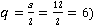; where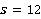is the number of seasons. This extracted part is added to the inadequate model obtained in (13).

The resulting expression gives the Mixed seasonal and Subset Fourier Model with Seasonal Harmonics shown below:

X_t=X ̅+a_1 Cosω_1 t+b_1 Sinω_1 t+a_2 Cosω_2 t+ b_2 Sinω_2 t +a_3 Cosω_3 t+b_3 Sinω_3 t+a_4 Cosω_4 t+b_4 Sinω_4 t+a_5 Cosω_5 t+b_5 Sinω_5 t+a_6 Cosω_6 t+b_6 Sinω_6 t-θ_1 a_(t-1)+ϕ_1 X_(t-1)-ϕ_2 X_(t-2)+ ϕ_3 X_(t-3)-ϕ_4 X_(t-4)+ϕ_5 X_(t-5)+e_t          (15)

Subjecting (15) to regression analysis using Minitab software gives the following estimated equation.

(X_t ) ̂=27.868+0.214Cosω_1 t-0.122Sinω_1 t+0.134Cosω_2 t+ 0.311Sinω_2 t-0.116Cosω_3 t-0.437Sinω_3 t+0.226Cosω_4 t-0.253Sinω_4 t-0.383Cosω_5 t+0.417Sinω_5 t+0.331Cosω_6 t+0.429Sinω_6 t-0.115a_(t-1)+0.412X_(t-1)-0.315X_(t-2)+ 0.341X_(t-3)-0.451X_(t-4)+0.338X_(t-5)                                           (16)

5.9. Diagnostic Checks of the Mixed seasonal and Subset Fourier Model with Seasonal Harmonics

5.9.1. Residual Variance and Autocorrelation Function

The residual variance obtained by fitting the model (16) is 4.32 and the residual autocorrelation function is displayed in figure 6 of the Appendix. Since there is no significant spike in the ACF plot; it means the residuals are serially uncorrelated. Under the null hypothesis of model adequacy, the residuals resemble the white noise process. Hence the model is adequate. Since amongst the models, the model (16) has the smallest variance; it performs better than the models (3) and (10).

5.9.2. Plot of the Actual and Estimated Series

The superimposed actual and estimated series plots (see figure 7 in the appendix) shows a strong positive correlation between them. This is clearly seen as the two plots are strongly interwoven and move in the same direction. Thus, the model gives a good fit to the data.

6. Discussion and Conclusion

In statistics, it is always advisable to choose a parsimonious model with the best fit. As highlighted earlier, fitting the complete Fourier series in this work will involve not less 60 orthogonal trigonometric functions. Interestingly, the mixed model in (11) has reduced the computational burden to less than 20 terms and has shown to fit well to the data. This has provided an advantage over the Fourier time series model in (7).

Secondly, it has been noted that the model with the least AIC and BIC values during model identification test is the best model to be used in the analysis, . However, it is clear in this work that this is not necessarily so. A model with minimum AIC and BIC can violate the assumption of model adequacy. Finally, it is believed that this research has offered special method of obtaining a parsimonious model in periodic time series.

Appendix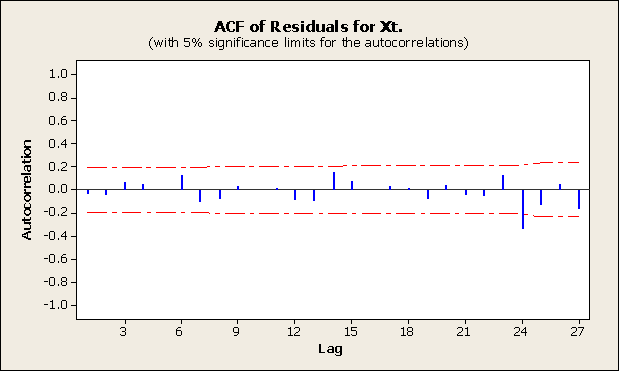Figure 4. Residual ACF of the SARIMA model.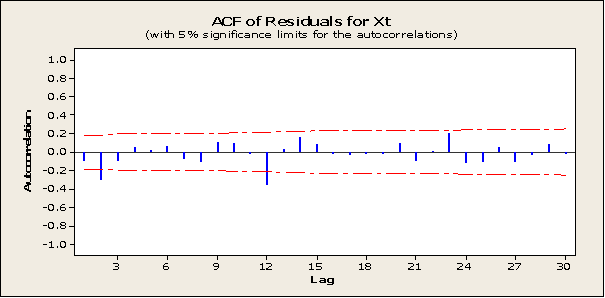Figure 5. Residual ACF of the Subset Fourier Series with Seasonal Harmonics model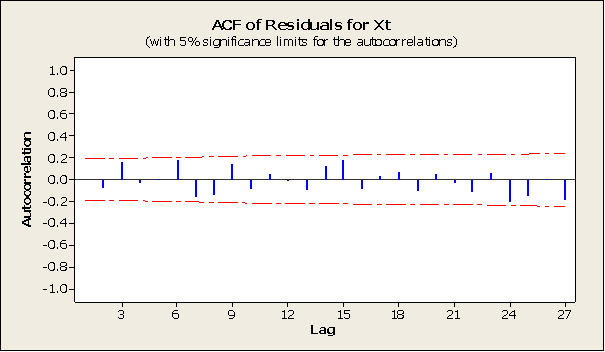Figure 6. Residuals ACF of the Mixed seasonal and Subset Fourier Modelwith  Seasonal Harmonics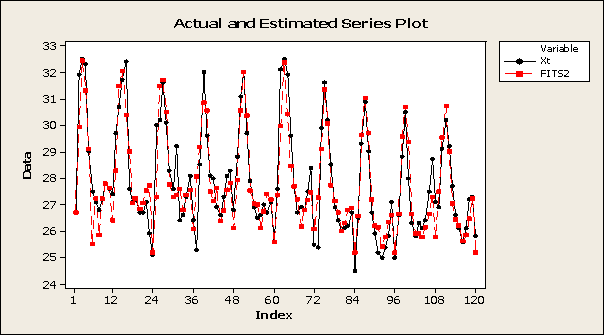Figure 7. Actual and Estimates plots of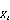.

References

1. Abdul-Aziz, E., John, P. and Keneth, T. (2013). Modelling and Forecasting Rainfall Pattern in GhanaAs a Seasonal ARIMA Process: The Case of Ashanti Region. International Journal of Humanities and Social Science.Vol 3. No. 3.
2. Beldjilali, E. P; Bello, K., Ahis, E. and Oge, H. (2016); Prediction of Ozone Concentrations According to the Box-Jenkins Methodology for Assekrem Area. Applied Ecology and Environmental Sciences. Vol. 4, No. 2, 2016 pp48-52. http://pubs.scie.com/aees/4/2/3.
3. Ekpenyong, E. J; Omekara, C. O. and Akpan, S. J. (2014). Modeling the Nigerian Inflation Rates Using Periodogram and Fourier Series Analysis Methods. The Nigerian case. International journal of African and Asian studies-(vol. 4 2014).
4. Ekpenyong, E. J and Omekara, C. O (2008). Application of Fourier Series Analysis to Modelling Temperature Data of Uyo Metropolis. Global Journal of Mathematical Sciences, Vol. 7 (1), 5-13. ISSN: 1596-6208.
5. Gurudeo, A. T. and Mahbub, I. (2010). Time series analysis of rainfall and temperature interaction in coastal catchments. Journal of Mathematics and Statistics 6 (3): 372-380, 2010. ISSN: 1549-3644.
6. Ibrahim, K., Teddy, S. and Yakubu, H. (2011). Modelling of Sokoto Daily Average Temperature: A Fractional Integration Approach.
http://www.ajol.info/index.php/njbas/index Nigerian Journal of Basic and Applied Science (2011), 19 (1): 21- 30 ISSN 0794-5698.
7. Liu L. M, Hudak, G., Box G. E. P., Muller M. E. and Tiao, G. C. (2014). Short-Term Forecasting of Temperature Driven Electricity Load Using Time Series and Neural Network Model. Journal of Clean Energy Technologies, vol 2, No. 4, pp 327-37, October 2014.
8. Peter, R. (2005). Time series modelling of global mean temperature for managerial decision-making. Journal of environmental management impact factor: 2. 72. DOI: 10.1016/j.jenvman. 2005. 01.008. source: pubmed.
9. Song, T. S., Petre, S. and Randolph, L. M. (2014). Introduction to Spectral Analysis. ISBN: 0-13-258419-0. Prentice Hall Upper saddle River, New Jersey 07458.
10. Wakaura M. and Ogata Y. (2007); A Time Series Analysis on the seasonality of air temperature anomalies. Meteorol. Appl. 14: 425-434(2007).
www.interscience.wiley.com
. DOI: 10.1002/met.41
.

 Contents 1. 2. 3. 3.1. 3.2. 3.3. 3.4. 3.5. 3.6. 3.7. 3.8. 3.9. 3.10. 4. 4.1. 4.2. 4.3. 5. 5.1. 5.2. 5.3. 5.4. 5.5. 5.6. 5.7. 5.8. 5.9. 6.
Article ToolsAbstractPDF(281K)